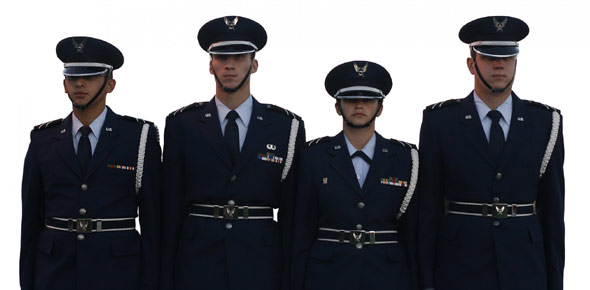# 2A251 Volume 5 UREs

100 Questions | Total Attempts: 85Settings• 1.
If a radar echo returnes after 310 microseconds, how far away is the target?
• A.

10 miles

• B.

25 miles

• C.

50 miles

• D.

75 miles

• 2.
In which radar system does the transmitter produce a signal that regularly changes over a known range of frequencies?
• A.

Beacon

• B.

Frequency-shift

• C.

Pulse-modulation

• D.

Frequency-modulation

• 3.
In a pulse-modulation radar system, the transmitter does not interfere with the receiver. This is possible because the transmitter
• A.

Is turned off after each pulse.

• B.

Shifts the frequency of the pulse

• C.

Transmits on a different frequency

• D.

Uses a different antenna than the receiver

• 4.
Which radar system is based on the Doppler principle?
• A.

Beacon

• B.

Frequency-shift

• C.

Pulse-modulation

• D.

Frequency-modulation

• 5.
In a pulse-modulation radar system, a high pulse repetition frequency radar limits range by
• A.

Using less power

• B.

Using more power

• C.

Shortening listening time

• D.

Lengthening listening time

• 6.
Which component in a pulse-modulation radar set supplies the voltages that operate the other radar components?
• A.

Antenna

• B.

Indicator

• C.

Transmitter

• D.

Power Supply

• 7.
Which component in a pulse-modulation radar system controls the operation of the entire radar set?
• A.

Antenna

• B.

Indicator

• C.

Transmitter

• D.

Synchronizer

• 8.
Where does the radar transmitter in a pulse-modulation radar system send a small amount of energy for use as a time reference?
• A.

Antenna

• B.

• C.

Indicator

• D.

Power Supply

• 9.
The minimum range of a pulse-modulation radar system is determined by the pulse
• A.

Shape

• B.

Duration

• C.

Recurrence time

• D.

Repetition frequency

• 10.
As the pulse duration of a radar system increases, what will happen to the systems minimum and maximum range?
• A.

Minimum range will increase, maximum range will increase

• B.

Minimum range will increase, maximum range will decrease

• C.

Minimum range will decrease, maximum range will decrease

• D.

Minimum range will decrease, maximum range will increase

• 11.
The maximum range of a pulse-modulation radar system primarily depends on
• A.

Pulse shape

• B.

Power output

• C.

Pulse recurrence time

• D.

Pulse repitition frequency

• 12.
In a frequency-shift radar system, the Doppler principle makes it possible to instantaneously sense and measure
• A.

Altitude and ground track

• B.

Target elevation and speed

• C.

Groundspeed and drift angle

• D.

• 13.
In a frequency-shift radar system, what is the relationship between a transmitter/receiver(T/R) and an aircraft when the received frequency is lower that the transmitted frequency?
• A.

They are a constant distance away from each other

• B.

The aircraft is not reflecting any of the radar pulses

• C.

The aircraft is flying toward the T/R

• D.

The aircraft is flying away from the T/R

• 14.
If the operating frequency is 100m megahertz (MHZ) with a wavelength of 3 meters, then the frequency determining wall of the waveguide would be
• A.

.9 meters

• B.

1.5 meters

• C.

2.1 meters

• D.

2.7 meters

• 15.
The function of a dummy load in search and weather radar is to
• A.

• B.

• C.

Prevent standing waves

• D.

Simulate targets

• 16.
What component is used to couple radio frequency energy between the waveguide and test equipment
• A.

Feedhorn

• B.

Duplexer

• C.

• D.

Directional Coupler

• 17.
What is triggered when the AS-653C Radar Antenna Reflector rotates through the aircraft lubber line?
• A.

Bearing Circuit

• B.

Tilt control circuit

• C.

Stabilization circuit

• D.

• 18.
Which component in the AN/APN-59 Radar System furnishes both pitch and roll drive signals to the antenna stabilization motors?
• A.

AS-653C

• B.

RT-289G

• C.

AM-853B

• D.

CN-221A

• 19.
What component in the AN/APN-59 Radar System provides stabilized pitch and roll reference data for control of antenna orientation, despite changes in aircraft altitude?
• A.

CN-221A

• B.

RT-289G

• C.

AM-853B

• D.

C-1242A

• 20.
What is the maximum height that the combined altitude radar altimeter subsystem computes aircraft altitude above ground level?
• A.

5,000 feet

• B.

25,000 feet

• C.

35,000 feet

• D.

50,000 feet

• 21.
When power is applied to the combined altitude radar altimeter, what is shown on the digital display?
• A.

All eights

• B.

All dashes

• C.

The word INIT

• D.

The word TEST

• 22.
What is/are the function(s) of the SET knob located on the combined altitude radar altimeter indicator?
• A.

Used as an on/off control

• B.

Used as an on/off control and to set the VALI indicator

• C.

Used as an on/off control and as a test button to check the system

• D.

Used as an on/off control, to set the VALI indicator, and as a test button to check the system

• 23.
What is the maximum altitude that the analog pointer and dial of the combined altitude radar altimeter can display?
• A.

5,000 feet

• B.

10,000 feet

• C.

25,000 feet

• D.

50,000 feet

• 24.
Which radar application is used to produce a pictorial map of the terrain?
• A.

Ground mapping

• B.

Terrain following

• C.

Terrain avoidance

• D.

• 25.
• A.

• B.

Ground mapping

• C.

Terrain following

• D.

Terrain avoidance

Related TopicsBack to top